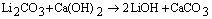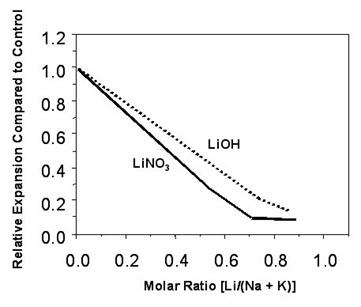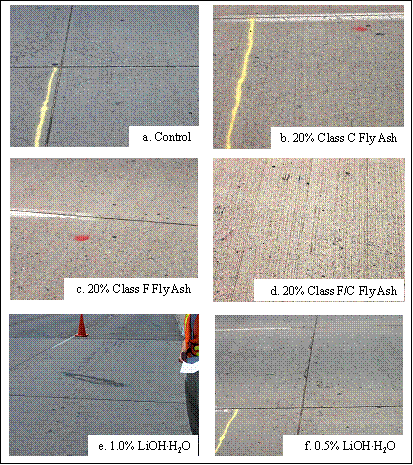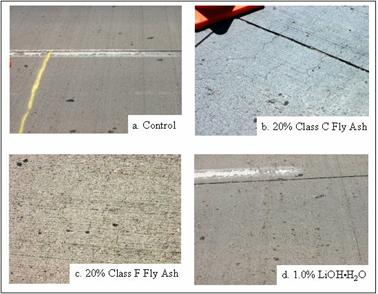U.S. Department of Transportation
1200 New Jersey Avenue, SE
Washington, DC 20590
202-366-4000

Federal Highway Administration Research and Technology
Coordinating, Developing, and Delivering Highway Transportation InnovationsThis report is an archived publication and may contain dated technical, contact, and link information
 Federal Highway Administration > Publications > Research Publications > Concrete Laboratory Publications > 06133 > 003.Cfm > The Use of Lithium to Prevent Or Mitigate Alkali-Silica Reaction in Concrete Pavements and Structures
 Publication Number: FHWA-HRT-06-133 Date: March 2007

# The Use of Lithium to Prevent Or Mitigate Alkali-Silica Reaction in Concrete Pavements and Structures

### Chapter 4. Using Lithium to Prevent ASR in New Concrete

#### 4.1 Laboratory Studies

In 1951, McCoy and Caldwell published data showing that the incorporation of various lithium compounds (LiCl, Li2CO3, LiF, Li2SiO3, LiNO3, and Li2SO4) in mortars (ASTM C227) containing highly reactive Pyrex ® glass could control damaging alkali-silica reaction provided the lithium was present in sufficient quantity. Although little work was conducted in the 40 years that followed the publication of this paper, there has been renewed interest in the use of lithium as an admixture, starting with the work conducted under the SHRP program (SHRP-C-343), and the last 15 years has seen the publication of numerous papers on the subject. Detailed reviews of the literature have been published elsewhere (Folliard et al., 2005; Feng et al., 2005).

The amount of lithium required to suppress expansion depends upon the form of lithium, the nature of the reactive aggregate, and the amount of alkali in the concrete. Many studies have shown that the expansion of concrete for a given aggregate depends on the amount of lithium relative to the amount of sodium plus potassium in the mortar or concrete mixture. This has led to the use of the molar ratio [Li]/[Na+K] for expressing the lithium dose in mortar and concrete mixtures, where [Li] is the number of moles of lithium and [Na+K] is the sum of the moles of sodium plus the moles of potassium present in the mixture.

McCoy and Caldwell's (1951) data showed that expansion was largely eliminated if the lithium-to-sodium-plus-potassium ratio was equal to or greater than 0.74; i.e., [Li]/[Na+K] ≥ 0.74. A number of recent laboratory studies have confirmed this finding, and [Li]/[Na+K] = 0.74 has become the "standard dose" for controlling ASR in concrete containing reactive aggregate.

At the time of writing this guideline, the only commercially available lithium compound for use as a concrete admixture is a solution containing 30 percent lithium nitrate (LiNO3). To achieve a molar ratio of [Li]/[Na+K] = 0.74 requires the addition of 4.6 L of 30-percent LiNO3 solution for every 1.0 kg of Na2Oe in the mixture (0.55 gal of solution for every 1.0 lb of Na2Oe), as shown in table 9.

Table 9. Example showing calculation of [Li]/[Na + K] molar ratio.

 Specific gravity of 30% LiNO3 solution = 1.2
Moles of lithium in 1 L of 30 % LiNO3 solution
 Mass of 1 L of 30% LiNO3 = 1,200 g

Alkali Molecular Weight Compound Molecular Weight

Li

7

LiNO3

69

Na

23

LiOH·H2O

42

K

39

Na2Oe

62

 Moles of lithium in 1 L of 30% LiNO3 solution Specific gravity of 30% LiNO3 solution = 1.2 Mass of 1 L of 30% LiNO3 = 1,200 g Mass of LiNO3 in 1 L = 30/100 x (1,200) = 360g Number of moles of LiNO3 = 360/69 = 5.217 moles Moles of sodium + potassium in 1 kg of Na2Oe Number of moles of Na2Oe in 1 kg of Na2Oe = 1,000/62 = 16.13 moles Number of moles of Na = 2 x 16.13 = 32.26 Volume of 30 % LiNO3 solution required for [Li]/[Na+K] = 0.74 Molar ratio for 1 L of 30% LiNO3 solution per 1 kg of Na2Oe = 5.217/32.26 = 0.162 Volume of 30% LiNO3 solution for molar ration of 0.74 = 0.740/0.162 = 4.6 L per 1 kg of Na2Oe 1 g = 0.035 ounce 1 Liter (L) = 0.26 gallon 1 kilogram (kg) = 2.20 lbs

Prior to the use of lithium nitrate, lithium hydroxide monohydrate (LiOH•H2O) was used in both laboratory testing and in a number of concrete structures (see section 4.3). To achieve a molar ratio of [Li]/[Na+K] = 0.74 requires the addition of 1 kg of LiOH•H2O for every 1 kg of Na2Oe in the mixture (1 lb LiOH•H2O for every 1 lb of Na2Oe).

LiOH•H2O is somewhat less effective than LiNO3 (see figure 16) as it introduces additional hydroxyl ions into the concrete. Furthermore, LiOH•H2O may exhibit a pessimum behavior with some aggregates, with low doses actually increasing expansion. Other lithium compounds such as Li2CO3 and LiCl may also increase the concentration of hydroxyl ions because of reaction with Ca(OH)2 in the paste as follows:(14)

Lithium nitrate does not produce this effect because of the high solubility of Ca(NO3)2.Figure 16. Relative expansion of concrete prisms (ASTM C1293) containing
lithium compounds and reactive siltstone aggregate (Thomas et al., 2000).

Although the "standard dose" of [Li]/[Na+K] = 0.74 appears to be sufficient to control expansion with a great many aggregates, it is not sufficient for all aggregate types (Lane, 2000; 2002; Durand, 2000; Tremblay, 2004), and higher doses are required. With some aggregates, a dose of 1.5 times the standard dose, i.e., [Li]/[Na+K] = 1.11, may still not be sufficient to suppress damaging ASR (Tremblay, 2004).

It would appear that lithium is more effective with rapidly reacting aggregates where the reactive phase is opal, chert, or volcanic glass, and less effective with slowly reacting aggregates which contain microcrystalline or strained quartz as the reactive phase. However, it is difficult to assess whether lithium will be effective or to determine the appropriate dose based purely on the mineralogy of the aggregates.

At this time, it is recommended that amount of lithium required to control expansion with a particular aggregate is determined by appropriate testing methods (see section 4.3).

#### 4.2 Field Applications

The first field application of lithium as an admixture in concrete containing reactive aggregate was in the construction of an experimental pavement in Albuquerque, NM, in 1992. Two local sources of highly reactive sand and gravel were used together with low-alkali cement (0.55 percent Na2Oe) and a range of preventive measures, which included lithium hydroxide monohydrate. Two doses of lithium were used; these were 0.5 percent and 1.0 percent LiOH•H2O by mass of cement, which yielded lithium-to-sodium-plus- potassium molar ratios of [Li]/[Na+K] = 0.67 and 1.34 (i.e., 91 percent and 182 percent of the standard dose), respectively. Figures 17 and 18 show selected sections of this pavement after 12 years of service. As shown in figure 17, cracking has occurred in the control section, the section with 20 percent Class C fly ash, the section with 20 percent of the blended Class F plus Class C fly ash, and in the section with 0.5 percent LiOH•H2O (lower dose lithium: [Li]/[Na+K] = 0.67). The section with the higher lithium dose ([Li]/[Na+K] = 1.34) is still performing well. These sections were constructed with the aggregate from the Shakespeare pit that contained mixed volcanic aggregates. Figure 18 shows the section with the higher dose of lithium and the aggregate from the Placitas pit, in Albuquerque that also contained mixed volcanics and is also in good condition after 12 years. There is no formal monitoring program tracking the long-term behavior of these pavements, and the only evaluation has been periodic visual inspections conducted by members of the research team or others.

Since the early 1990s, lithium compounds have been used in a variety of concrete structures across the United States, and some of these have been described in detail by Folliard et al., 2003. There have been no reports of ASR in these structures at the time of writing this report.Figure 17. Photographs of 12-year-old pavement sections reactive aggregate
from Shakespeare pit in Albuquerque, NM (photos taken in 2004).Figure 18. Photographs of 12-year-old pavement sections
reactive aggregate from Placitas pit in Albuquerque, NM.

#### 4.3 Laboratory Testing To Determine the Amount of Lithium Required

To determine the amount of lithium required to control ASR with a specific aggregate, it is recommended that the combination of materials be tested using a modification of the concrete prism test (ASTM C1293). The only modifications necessary are to add the required amount of lithium nitrate solution to the mix water and to correct the total water content for the water contained in the lithium nitrate solution. The test should be conducted at a range of different lithium doses to determine the minimum amount required to control expansion (< 0.040 percent at 2 years) with the aggregate under consideration. Table 10 provides an example of calculation for concrete prism testing using a standard dose (i.e., [Li]/[Na+K] of 0.74) of LiNO3.

Table 10. Proportioning mixtures with lithium for the concrete prism test.

 Control Mixture 420 kg/m3 portland cement, NaOH added to mix water to raise cement alkalies to 1.25 percent: Total alkalis in concrete = 420 x 1.25/100 = 5.25 kg/m3 Na2Oe (15) Water-to-cement ratio, W/CM = 0.42 to 0.45 (16) Mixture with [Li] / Na + K] = 0.74 420 kg/m3 portland cement, NaOH added to mix water to raise cement alkalies to 1.25 percent: Total alkalis in concrete = 420 x 1.25/100 = 5.25 kg/m3 Na2Oe (17) Add: 30-percent LiNO3 solution at 4.6L per kg of Na2Oe = 4.6 x 5.25 = 24.15L   (18) Water-to-cement ratio, W/CM = 0.42 to 0.45 (19) include water in 30-percent LiNO3 solution. Calculate as follows: Mass of solution = volume x density = 24.15 L x 1.2 kg/L = 28.98 kg/m3 (20) Water present in solution = 70-percent of mass = 70/100 x 28.98 = 20.29 kg (21) This water should be included in the calculation of W/CM (i.e., it should be subtracted from the water content used in the control mixture).

The accelerated mortar bar test (ASTM C1260) cannot be used to evaluate lithium without significant modification. Immersion of the mortar bars in the high temperature alkaline solution overwhelms the mortar bar with alkali and changes the [Li]/[Na+K]. Proposed modifications have included adding lithium to both the mortar mixture and the soak solution using the same [Li]/[Na+K] ratio. However, there is currently little information available to confirm whether the lithium dose determined using a modified version of the accelerated mortar bar test is similar to that determined using the longer-term concrete prism test and field experience.

#### 4.4 Effect of Lithium on the Properties of Concrete

The use of lithium nitrate solution, at the levels of addition necessary to effectively control expansion due to ASR, has no adverse effect on the properties of fresh and hardened concrete, even at dosages in excess of 10 liters per cubic meter. The use of lithium nitrate may slightly enhance the workability of concrete (i.e., there is a small water-reducing effect) and lead to small decreases in the setting time (i.e., slight set-accelerating effect).